## The twice punctured torus

For the conference honoring the 60th birthday of Caroline Series (only a German wiki?!?), I was one of a handful asked to contribute pictures inspired by her work. First up is my contribution followed by a description. After that are a few more.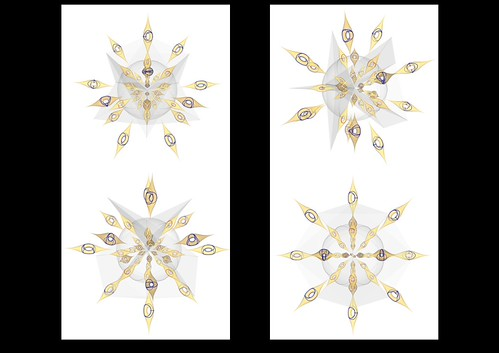(Hmmmm…. I guess I had transparency in the original background. Oh well.)

The projective measured lamination space of the twice punctured torus is homeomorphic to the three-sphere,$\mathcal{PML}(\Sigma_2) \cong S^3$. That’s a result of Thurston. Parker-Series , continuing work of Keen-Parker-Series , gives a triangulation of$\mathcal{PML}(\Sigma_2)$ consisting of 28 tetrahedra, 56 faces, 39 edges, and 11 vertices. Each vertex may be represented by a particular (isotopy class of an) unoriented simple closed curve while each tetrahedron is spanned by the projective weightings of a Birman-Series$\pi_1$-train track .

Two of these vertices correspond to natural North and South Poles of the three-sphere as they never occupy the same tetrahedron and the remaining 9 vertices with the 14 faces among them form a polyhedral two-sphere separating these poles.
We are thus able to display this triangulation of$\mathcal{PML}(\Sigma_2) \cong S^3$ in$\mathbb{R}^3$ by omitting the North Pole, placing the South Pole at the origin, choosing an embedding of the polyhedral$S^2$ enclosing the origin, and extending the remaining edges and faces radially. (Actually when shown in these pictures I used a round sphere while the vertices of the polyhedral sphere defined the radial edges.) At each vertex other than the poles we center a copy of$\Sigma_2$ with its corresponding simple closed curve; at a representative point within each tetrahedron we center of copy of$\Sigma_2$ with its corresponding fundamental train track. To keep the parametrization of each copy of$\Sigma_2$ consistent we first view$\Sigma_2$ as being skewered along a radial axis with one puncture heading towards the North Pole and the other towards the South Pole. Then we ask the model (using the Face Me function in SketchUp… looks like it’s now called Always Face Camera) to rotate each copy of$\Sigma_2$ along its axis so that its “front” maximally faces the viewer. This allows us to dynamically rotate the model while inferring the parametrization of each copy of$\Sigma_2$ by its radial orientation.

 J. R. Parker and C. Series, The mapping class group of the twice punctured torus, Groups: topological, combinatorial and arithmetic aspects}, London Math. Soc. Lecture Note Ser. 311 (2004), 405–496.

 L. Keen, J. R. Parker and C. Series, Combinatorics of simple closed curves on the twice punctured torus, Israel J. Math. 112 (1999), 719–749.

 J. S. Birman and C. Series, Algebraic linearity for an automorphism of a surface group, J. Pure and Applied Algebra 52 (1988), 227–275.

Generic train tracks for the tetrahedra:Simple closed curves for the vertices (sans the poles):The four pics of the full triangulation that make up the first picture: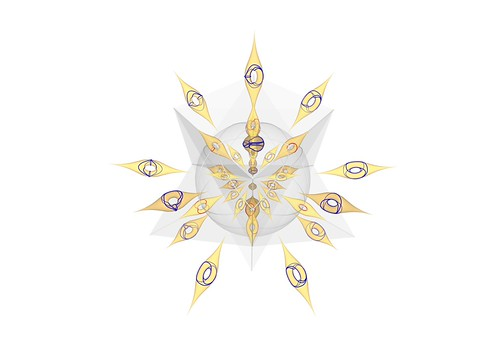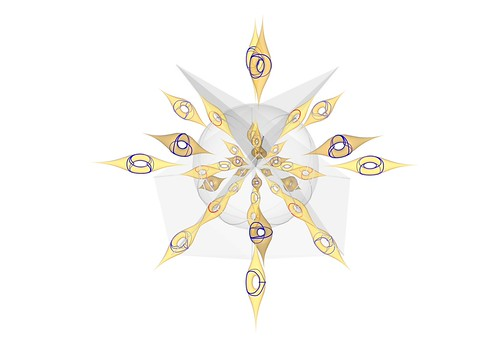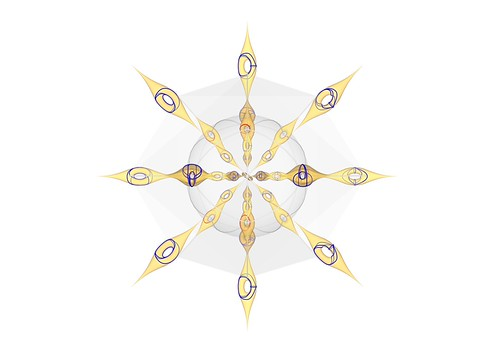The list of tetrahedra and vertices labeled with the Parker-Series notation: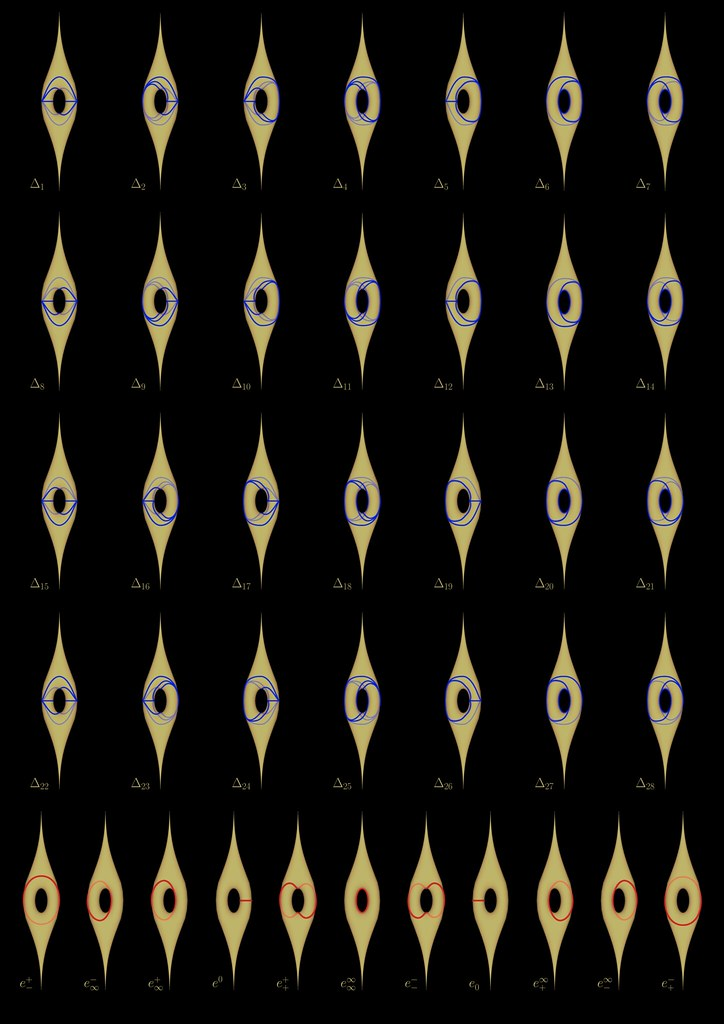And of course clicking on the pictures will take you to Flicker where you can view somewhat higher resolution versions.

~ by Ken Baker on July 29, 2011.

### One Response to “The twice punctured torus”

1. […] Ken Baker: The twice-punctured torus […]